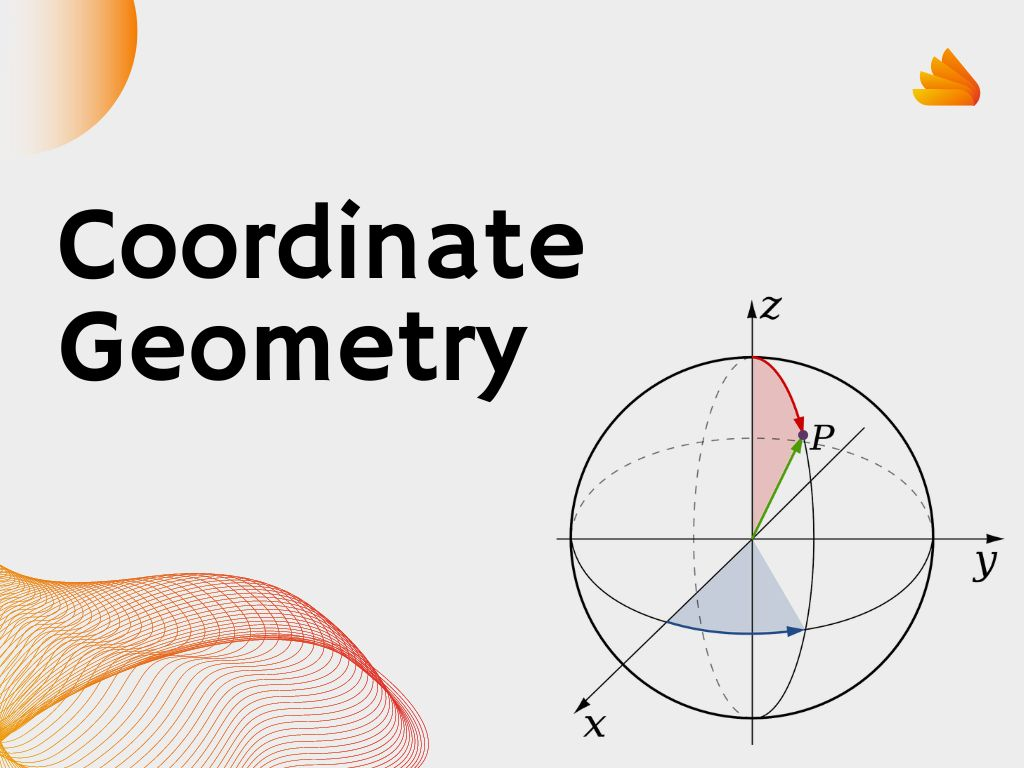## Coordinate Geometry Class 9th

Introduction In Class 9th, Before studying Coordinate Geometry (Analytical Geometry), We shall recall the number lines that how the number line is drawn, and how a number is represented on the number line. The number line shows positive and negative numbers in both directions. On the left side, negative numbers are written and on the …